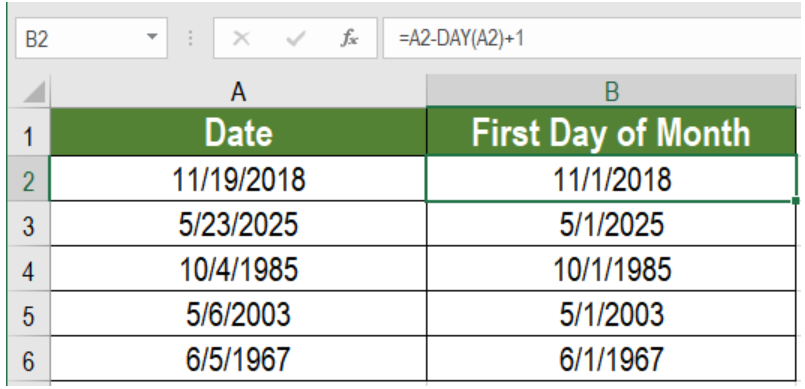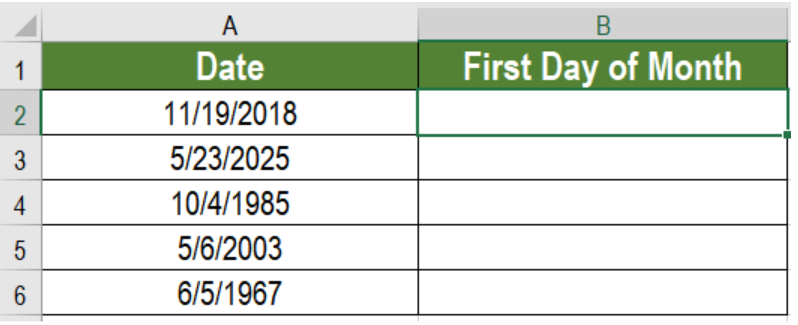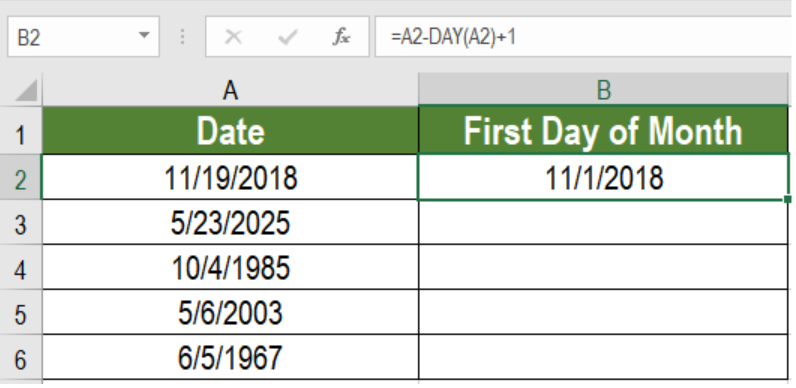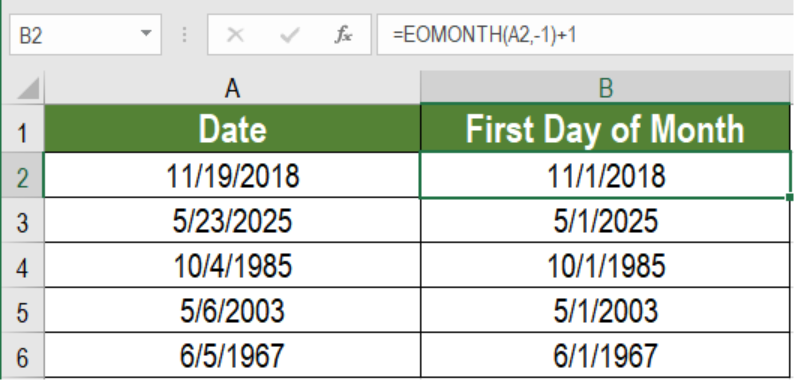Get instant live expert help with Excel or Google Sheets“My Excelchat expert helped me in less than 20 minutes, saving me what would have been 5 hours of work!”

#### Post your problem and you’ll get expert help in seconds.

Your message must be at least 40 characters
Our professional experts are available now. Your privacy is guaranteed.

# Get first day of month

The date is very important working on different financial and other worksheets. In many cases, we need to input the first day of a month. Microsoft Excel offers an easy fix to get the first day of the month. In this tutorial, we will learn how to get the first day of the month.Figure 1. Example of How to Get First Day of Month

## Syntax

`=date-DAY(date)+1`

## How this Formula Works

Here, the formula contains the DAY function in order to extract the first day of a month. Excel stores dates as serial numbers. The day function returns a number from 1 to 31 to represent the month’s days. Here, we subtract this number from the serial number and add 1. As a result, we get the first day of the month.

## Setting up Data

The following database uses some sample dates. Column A has these dates.Figure 2. The Sample Data Set

To get the first day of the months, we need to:

1. Go to cell B2. Click on it with the mouse.
2. Input the formula `=A2-DAY(A2)+1 `to the function box in B2.
3. Press Enter.Figure 3. Applying the Formula

1. Drag the formula from cells B2 to B6.

This will return the first day of the month in column B.

## Notes

We can use the EOMONTH formula to find the first day of the month as well. EOMONTH returns the last day of a month from a date. Here, we use the EOMONTH function to go to the last day of the previous month. Then, we add 1 to get the first day of the current month. To perform the previous example with the EOMONTH function, we need to use the formula `=EOMONTH(A2,-1)+1` in cell B2.Figure 4. Get First Day of Month Using EOMONTH

This will also show the first day of the month in column B.

Most of the time, the problem you will need to solve will be more complex than a simple application of a formula or function. If you want to save hours of research and frustration, try our live Excelchat service! Our Excel Experts are available 24/7 to answer any Excel question you may have. We guarantee a connection within 30 seconds and a customized solution within 20 minutes.

### Did this post not answer your question? Get a solution from connecting with the expert.Another blog reader asked this question today on Excelchat:
Solution examplesNeeding help expressing a date based on date range If Date = 01 OCT 2017 - 30 APR 2018 + 380 DAYS If Date = 01 MAY 2018 - 31 JUL 2018 + 270 DAYS If Date = 01 AUG 2018 - 30 SEP 2018 + 430 DAYS
Solved by E. J. in 14 minsNEED A FORMULA TO SHOW THE FOLLOWING: IF (Q3) IS AFTER TODAY & (R3) IS BLANK,THEN "BEHIND SCHEDULE". IF (R3) IS POPULATED THEN "COMPLETE". IF (Q3) IS
Solved by E. U. in 42 minsI need to modify my formula below to automatically the change cell color to yellow if the result in result in cell H3 is greater than 30. This formula is in cell H3 =IF(ISNUMBER(B3), TODAY()-B3, " ")
Solved by O. J. in 39 minsHOW CAN I COUNT NUMBERS PER DAY FOR A MONTH AS TOTAL?
Solved by F. F. in 16 minsHI GOOD DAY I NEED FORMULA ON EXCEL IF A IS 2499 B IS 20% AND IF A IS 2501 THEN B IS 30% HOW TO WRITE FORMULA FOR THIS
Solved by B. S. in 20 mins## Subscribe to Excelchat.coAnother blog reader asked this question today on Excelchat: